ZhouYongyi's Personal Blog# 吴恩达《机器学习》课程学习笔记（二）—— 单变量线性回归

## 一、模型描述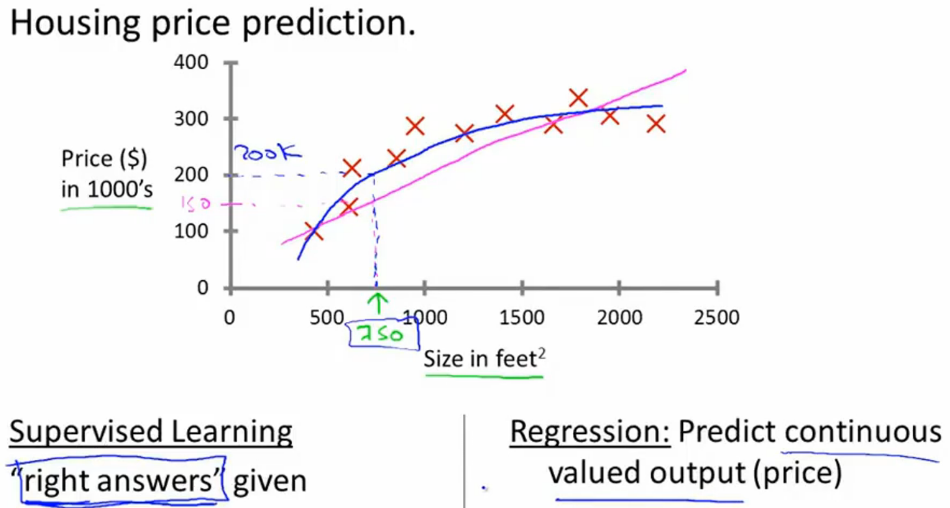• m=用于训练的样本数
• $$x^i =$$第i个训练样本“输入”变量/特征量
• $$y^i =$$​第i个训练样本“输出”变量/特征量

$h_{\theta }=\theta_{0}+\theta_{1}x \space\space\space\space\space\space\space\space\space\space\space\space\space\space\space(1.1)$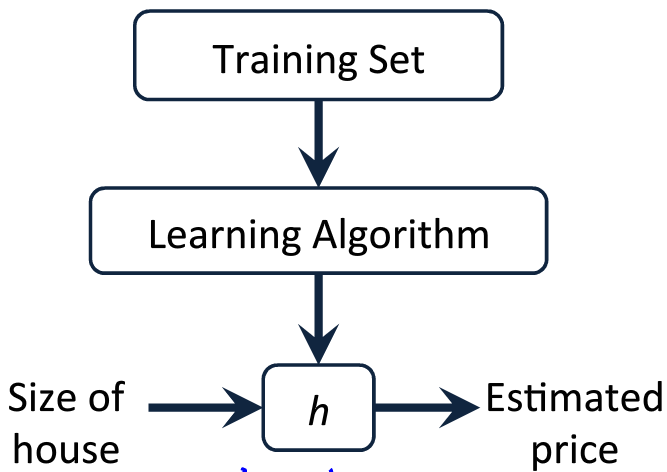## 二、代价函数（Cost function）

$J(\theta_{0},\theta_{1})=\frac{1}{2m}\sum_{i=1}^{m}(h_{\theta}(x^{(i)})-y^{(i)})^2 \space\space\space\space\space\space\space\space\space\space\space\space\space\space\space(2.1)$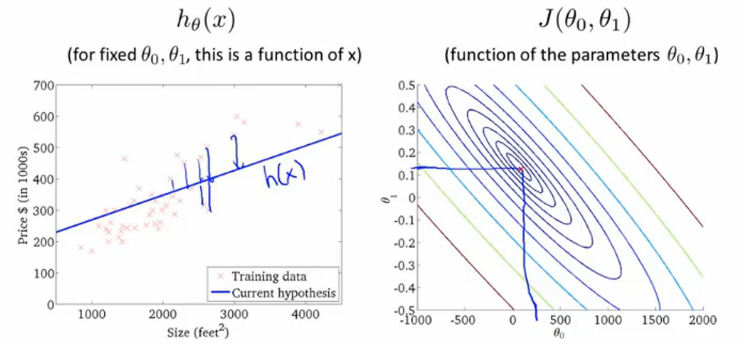• 从某一对​$$\theta_{0},\theta_{1}$$​出发
• 不断尝试改变$$\theta_{0},\theta_{1}$$，使得$$J（\theta_{0},\theta_{1}）$$ 减小，逐步逼近最小值（迭代）
• 改变的策略：每一次改变的方向，取当前位置梯度下降的方向：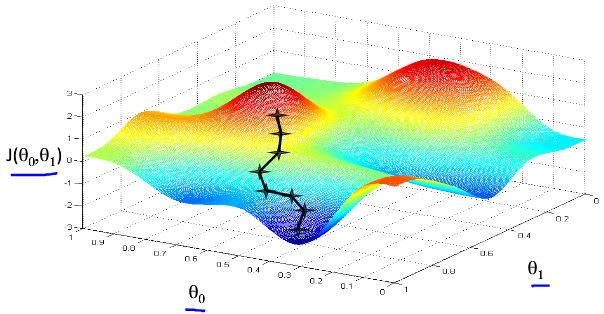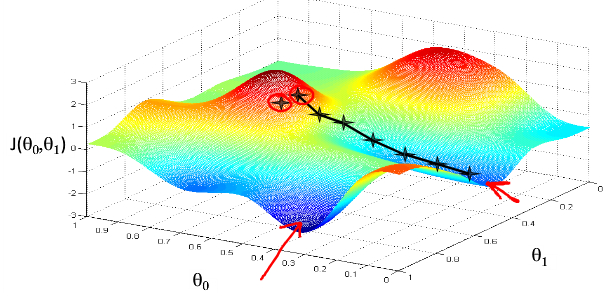$\theta_{j}:=\theta_{j}-\alpha\frac{\partial }{\partial \theta_{j}}J(\theta_{0},\theta_{1}) \space\space\space\space\space\space\space\space\space\space\space\space\space\space\space(3.1)$

$\theta_{0}:=\theta_{0}-\alpha\frac{\partial }{\partial \theta_{0}}J(\theta_{0},\theta_{1}) \space\space\space\space\space\space\space\space\space\space\space\space\space\space\space$

$\theta_{1}:=\theta_{1}-\alpha\frac{\partial }{\partial \theta_{1}}J(\theta_{0},\theta_{1})x^{(1)} \space\space\space\space\space\space\space\space\space\space\space\space\space\space\space$

• $temp0:=\theta_{0}-\alpha\frac{\partial }{\partial \theta_{0}}J(\theta_{0},\theta_{1})$

• $temp1:=\theta_{1}-\alpha\frac{\partial }{\partial \theta_{1}}J(\theta_{0},\theta_{1})$

• $\theta_{0}:=temp0$

• $\theta_{1}:=temp1$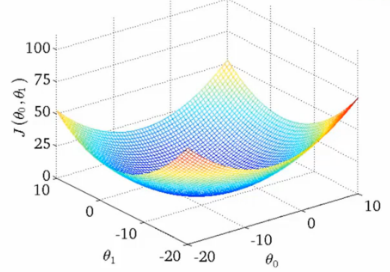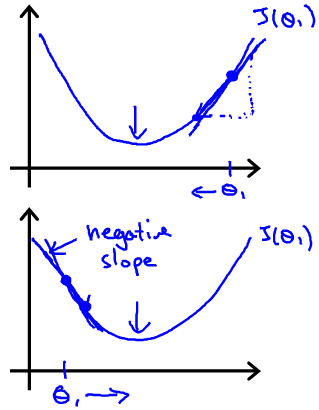• 当θ大于最小值时，导数为正，那么迭代公式​$$\theta:=\theta-\alpha\frac{\partial }{\partial \theta}J(\theta)$$​里，θ减去一个正数，向左往最小值逼近；
• 当θ小于最小值时，导数为负，那么迭代公式​$$\theta:=\theta-\alpha\frac{\partial }{\partial \theta}J(\theta)$$​里，θ减去一个负数，向右往最小值逼近；##### xky##### zhouyongyi##### zhouyongyi

theta = zeros(2, 1); % initialize fitting parameters

$${}$$